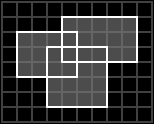### Problem Statement

NOTE: This problem statement contains an image that may not display properly if viewed outside of the applet.

Given a list of two-dimensional rectangles, compute the area of their union. For example, the union of the three rectangles shown in the figure below:cover an area of 35 units.

The list of rectangles will be given as a String[], where each element describes one rectangle. Each String will be formatted as 4 space-separated integers with no leading zeros, giving the coordinates of the left, bottom, right, and top of the rectangle (in that order). The three rectangles shown above would be given as:

```    { "1 3 5 6",
"3 1 7 5",
"4 4 9 7" }
```

### Definition

 Class: BoxUnion Method: area Parameters: String[] Returns: int Method signature: int area(String[] rectangles) (be sure your method is public)

### Constraints

-rectangles will contain between 1 and 3 elements, inclusive.
-Each element of rectangles will be formatted as described in the problem statement.
-For each rectangle, the left coordinate will be less than the right coordinate and the bottom coordinate will be less than the top coordinate.
-All coordinates will be between 0 and 20000, inclusive.

### Examples

0)

 `{ "200 300 203 304" }`
`Returns: 12`
 A single rectangle with area 12.
1)

 ```{ "0 0 10 10", "20 20 30 30" }```
`Returns: 200`
 Two disjoint rectangles, each of area 100.
2)

 ```{ "0 500 20000 501", "500 0 501 20000" }```
`Returns: 39999`
 These two rectangles intersect at a single point.
3)

 ```{ "4 6 18 24", "7 2 12 19", "0 0 100 100" }```
`Returns: 10000`
 The third rectangle completely overlaps the first two.
4)

 ```{ "1 3 5 6", "3 1 7 5", "4 4 9 7" }```
`Returns: 35`
 This is the example from the problem statement.
5)

 ```{ "0 0 20000 20000", "0 0 20000 20000", "0 0 20000 20000" }```
`Returns: 400000000`

#### Problem url:

http://www.topcoder.com/stat?c=problem_statement&pm=4463

#### Problem stats url:

http://www.topcoder.com/tc?module=ProblemDetail&rd=6536&pm=4463

legakis

#### Testers:

PabloGilberto , lbackstrom , brett1479

#### Problem categories:

Geometry, Simple Math